# The Crystal Systems

Posted by Vidal Dias on

One of six (or seven) group classifications of a crystalline mineral according to the symmetry of its crystal faces. Each species occurring in only one of those systems. They are cubic, tetragonal, hexagonal (trigonal), orthorhombic, monoclinic, and triclinic.

### Crystalline Solids

The majority of solids are crystalline in nature. A crystal is a substance in which the particles are arranged in an orderly, repeating, three-dimensional pattern. Particles of a solid crystal may be ions, atoms, or molecules, depending on the type of substance. The three-dimensional arrangement of a solid crystal is referred to as the crystal lattice . Different arrangements of the particles within a crystal cause them to adopt several different shapes.

### Crystal Systems

Crystals are classified into general categories based on their shapes. A crystal is defined by its faces, which intersect with one another at specific angles, which are characteristic of the given substance. The seven crystal systems are shown below, along with an example of each. The edge lengths of a crystal are represented by the letters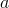,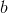, and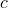. The angles at which the faces intersect are represented by the Greek letters $\alpha$, $\beta$, and $\gamma$. Each of the seven crystal systems differs in terms of the angles between the faces and in the number of edges of equal length on each face.

Seven Basic Crystal Systems and an Example of Each
Crystal System Diagram Example

Cubic

a = b = c; α = β = γ = 90°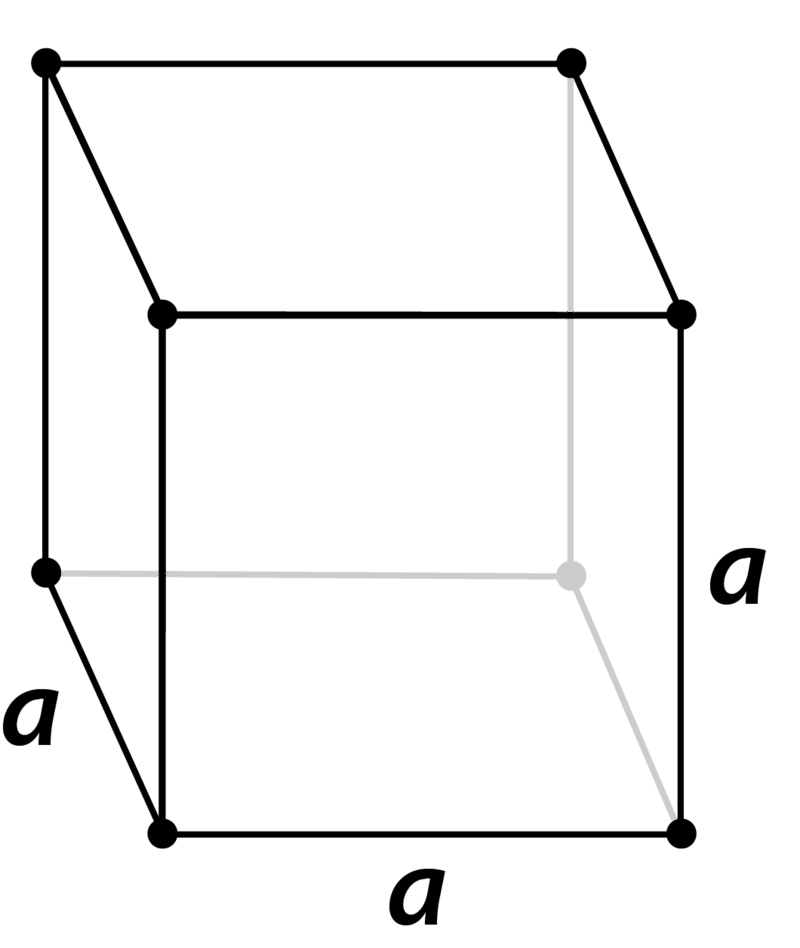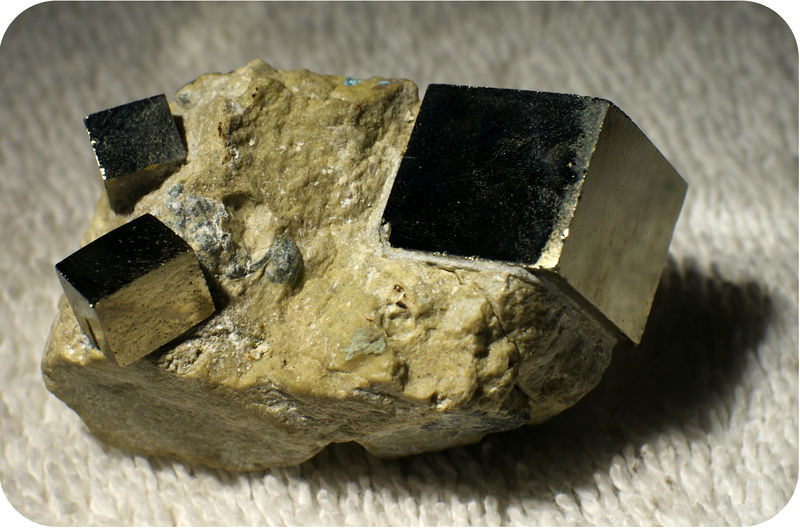Pyrite

Tetragonal

a = b ≠ c; α = β = γ = 90°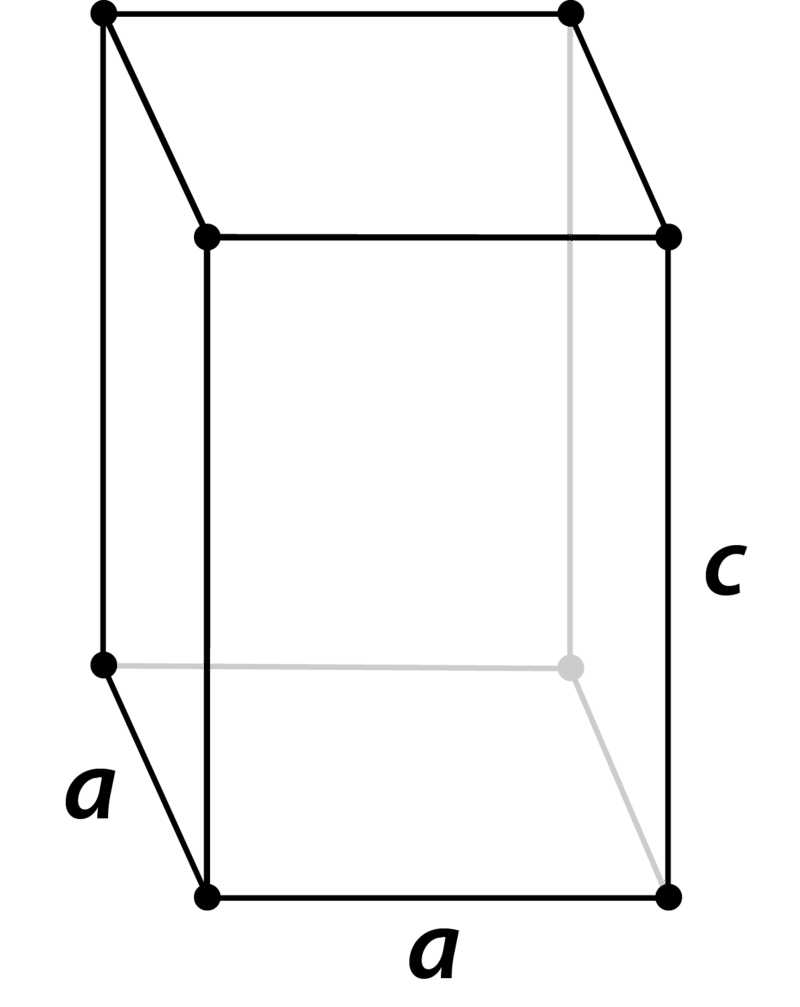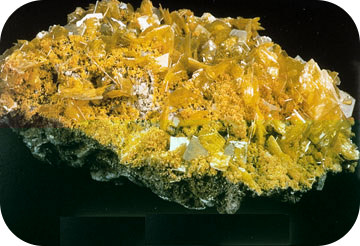Wulfenite

Orthorhombic

a ≠ b ≠ c; α = β = γ = 90°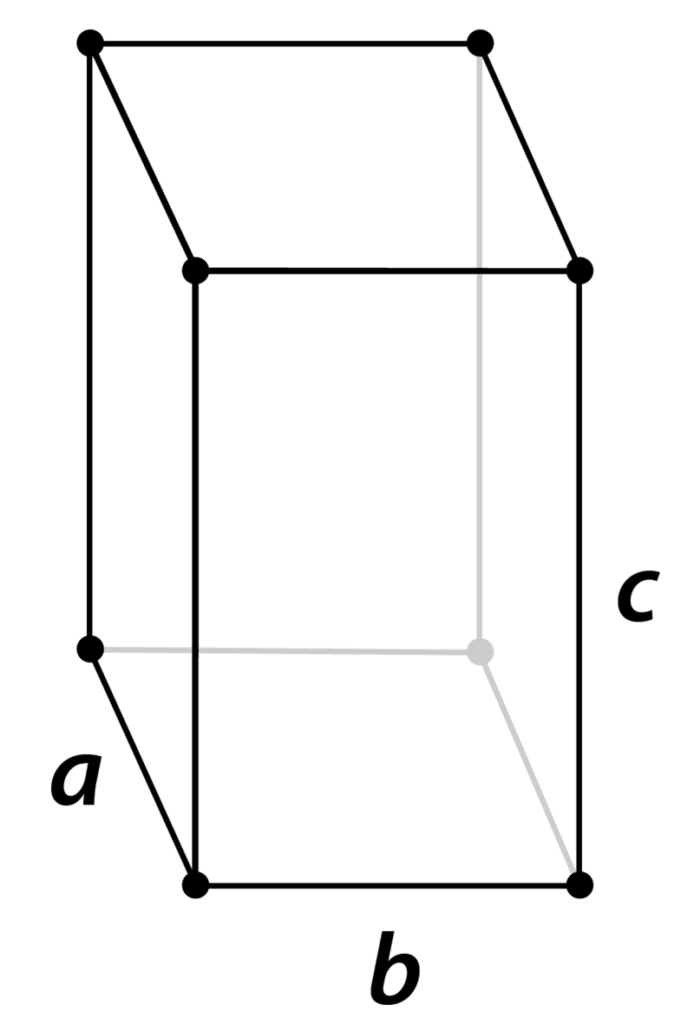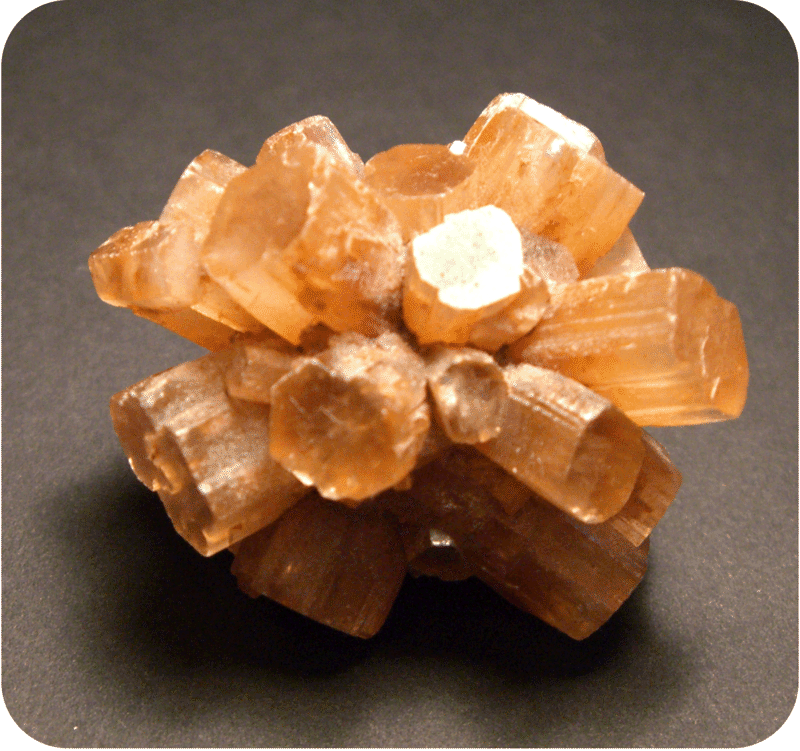Aragonite

Monoclinic

a ≠ b ≠ c; α ≠ 90° = β = γ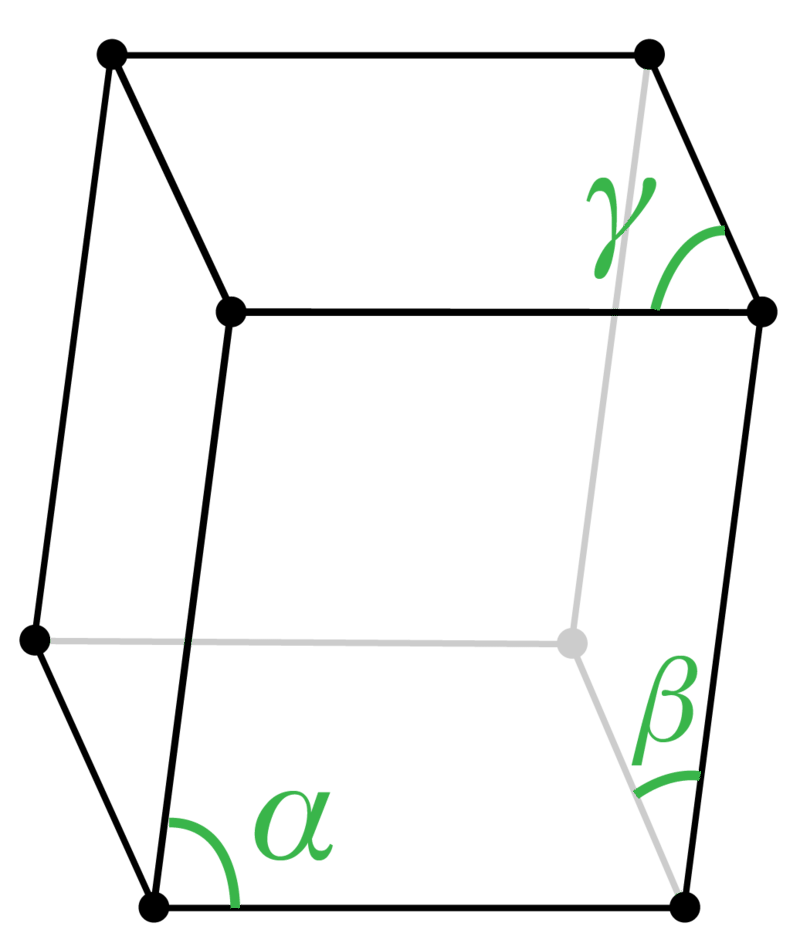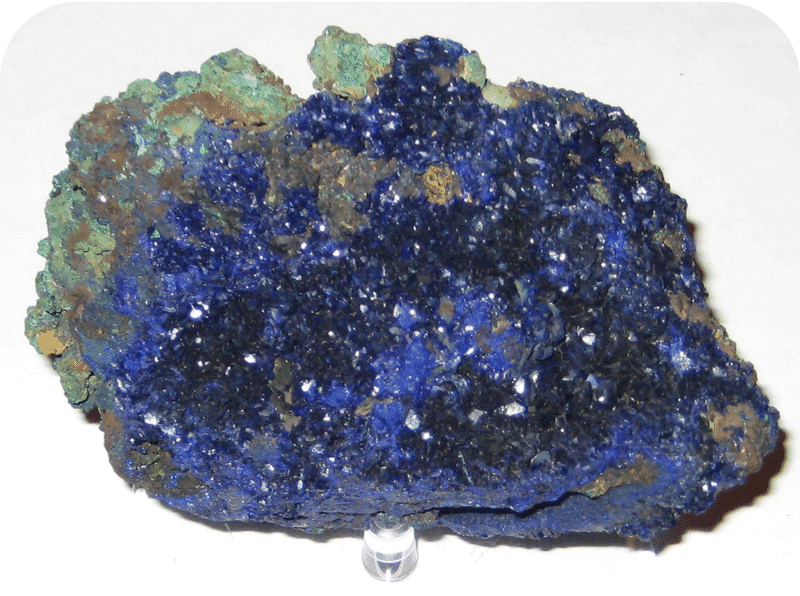Azurite

Rhombohedral

a = b = c; α = β = γ ≠ 90°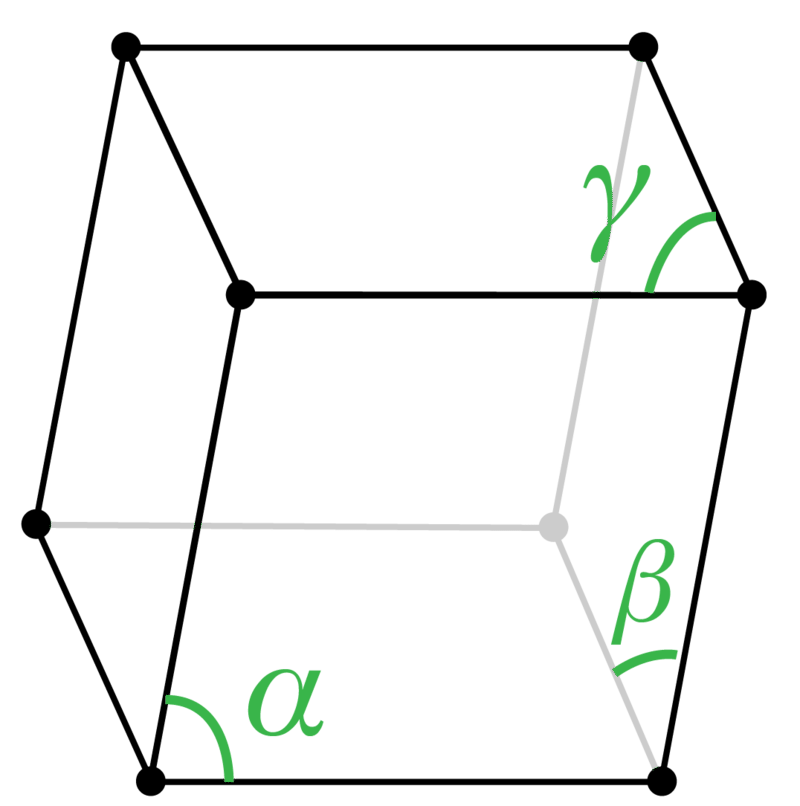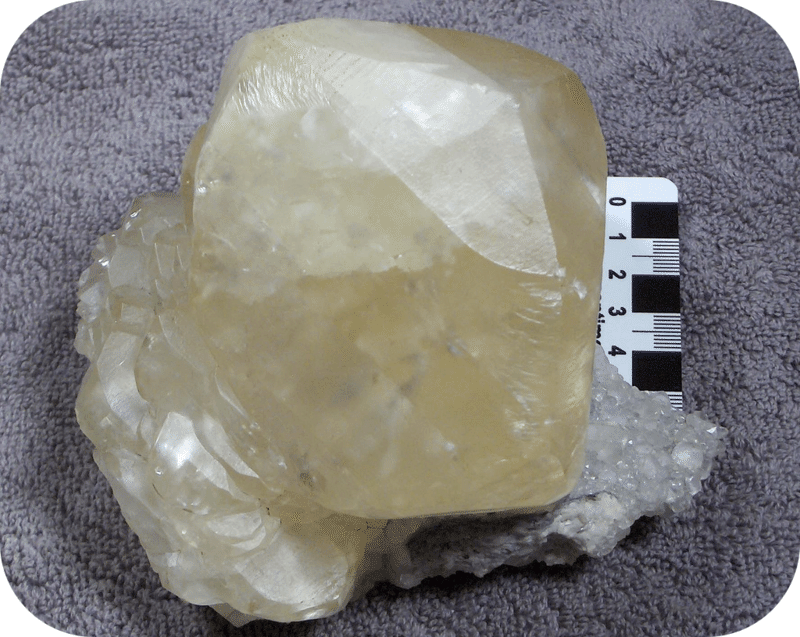Calcite

Triclinic

a ≠ b ≠ c; α ≠ β ≠ γ ≠ 90°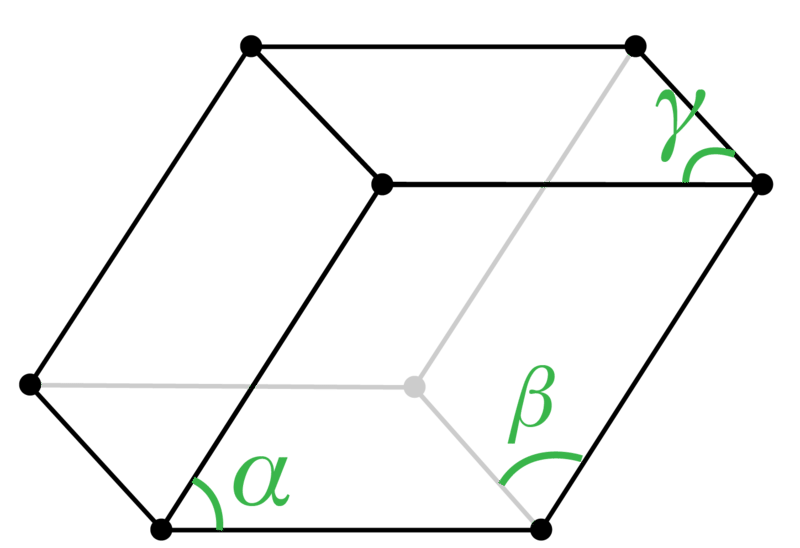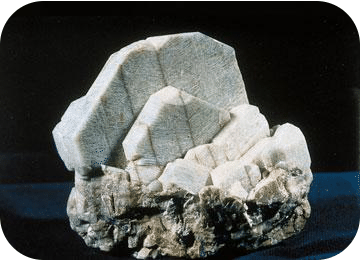Microcline

Hexagonal

a = b ≠ c; α = β = 90°, γ = 120°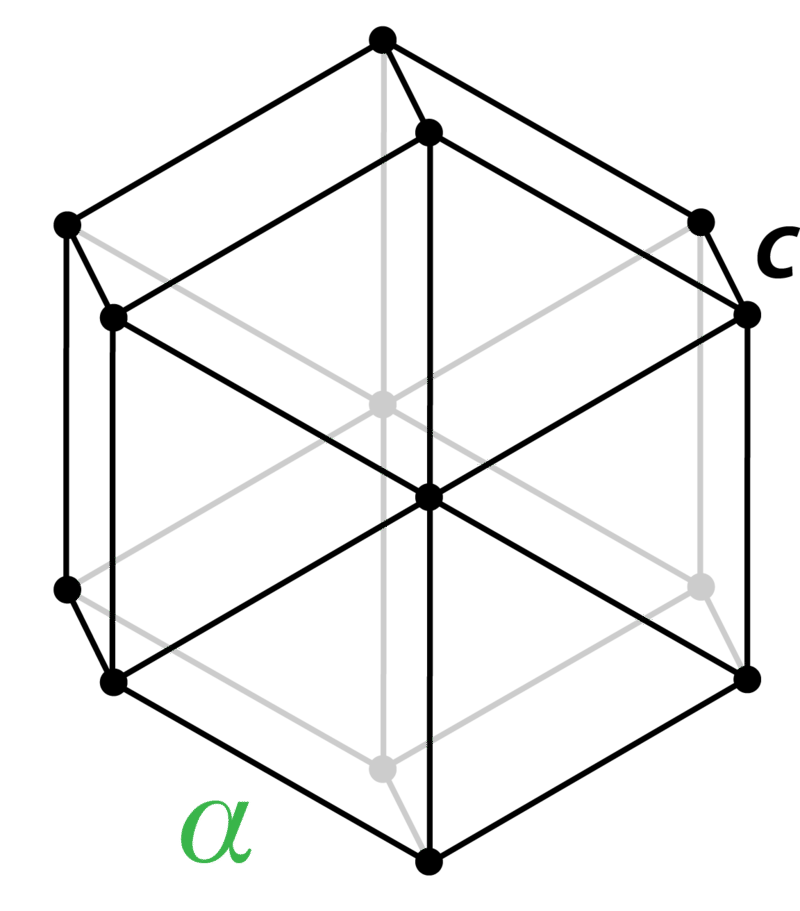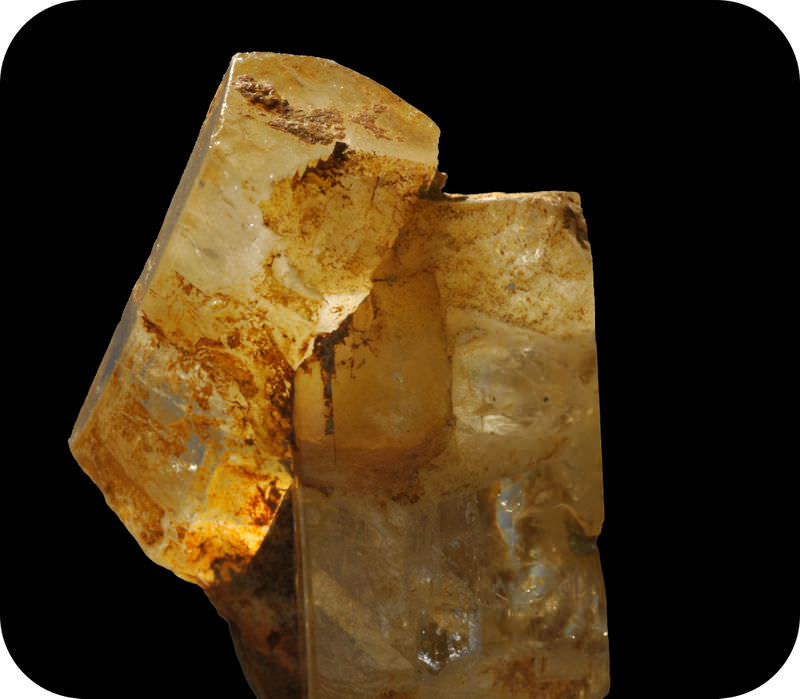Beryl

### Unit Cells

#### Summary

• A crystal is a substance in which the particles are arranged in an orderly, repeating, three-dimensional pattern.
• The crystal lattice is the three-dimensional arrangement of a solid crystal.

References: Request sources by emailing me@iamvoyager.life